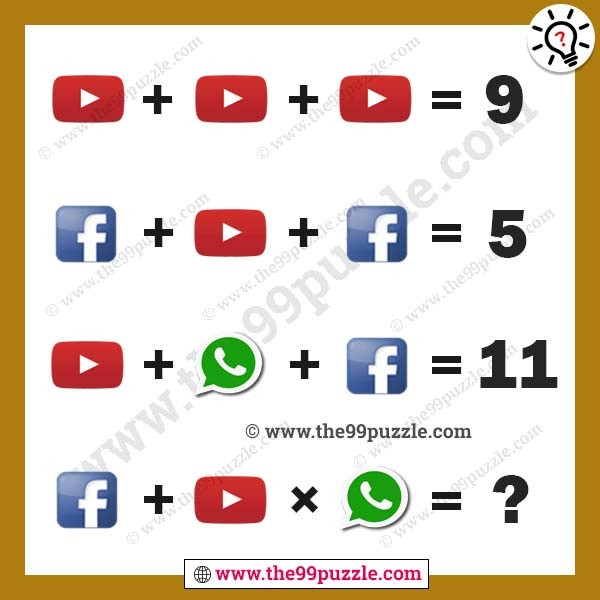# Social media picture puzzles with answer – Puzz165

Social media picture puzzles with answers (Youtube, Facebook, whatsapp). These picture puzzles are challenging for people. Can you calculate the math equation picture puzzle?3 + 3 + 3 = 9

1 + 3 + 1 = 5

3 + 7 + 1 = 11

1 + 3 × 7 = 22